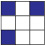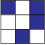# User Forum

Subject :NSO    Class : Class 3

Find the odd one out.

ABCDd

Class : Class 3

Class : Class 6
why?

## Ans 3:

Class : Class 3
Answer is asymmetrical figure, because all the other figures are symmetrical.

## Ans 4:

Class : Class 3
The images given in Options A, B and D are symmetrical images. That means all three have at least one line of symmetry. Line of symmetry is any line drawn through the image which divides the image into two exactly equal parts, and these parts are mirror images of each other. A.The part above the red line (line of symmetry) and the 7part below the red line are mirror images of each other.7410`1 B.The parts on both sides of the red line (line of symmetry) are mirror images of each other. C.There can be no line of symmetry that can divide this image into two equal parts which are mirror images of each other. D.The part on the left side of the red line (line of symmetry) and the part on the right side of the red line are mirror images of each other. Hence, Option C is the odd one out.

Class : Class 5
why

Class : Class 3
understood

Class : Class 3
ANS should be a

## Ans 8:

Class : Class 4
Figure A can be folded horizontally, figure B can be folded diagonally, figure D can be folded vertically to get their mirror images whereas figure C is asymmetric, so the odd one out is figure C

Class : Class 4

## Ans 10:

Class : Class 3
nonsense question